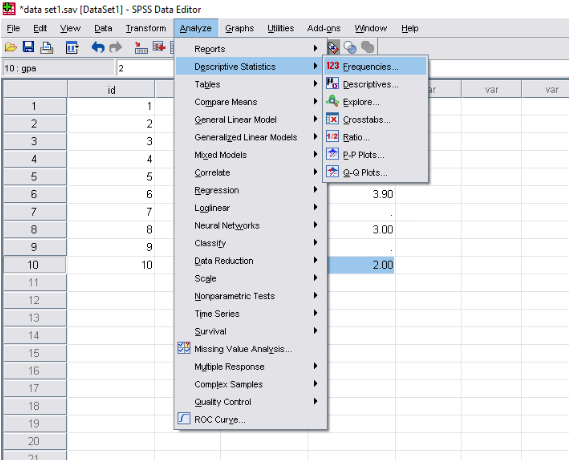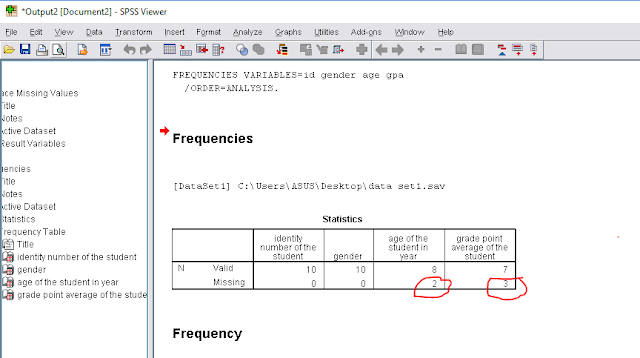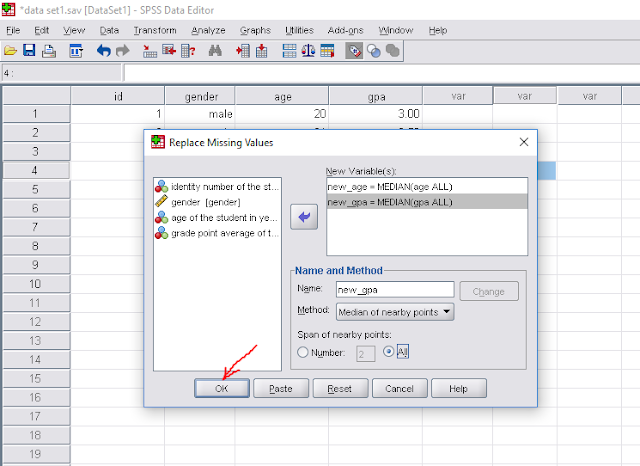# How to analyze data using SPSS (part-7), Finding missing values, Replacing missing values, Coding missing values in SPSS

Missing values in SPSS:

How can we find missing values?

-To find the missing values we have to go "Menu bar" and click on ,
"Analyze"→"Descriptive statistics"→"Frequencies"
Then there open a new box named "Frequencies" , now  we have to double click on the every variable and click on "ok".Finding missing values

↓

Now we can see the output window with frequencies of the variables.
If we have any missing value , we can see the number of missing in "missing sector"Finding missing values

Now we have to replace the missing values. For replacing the missing values we have go to "Menu bar" and click on "Transform"→"Replace missing values", then there open a new box named "Replace missing values" and double click on the variable which contains missing value. for example
"age of the students in year" and "grade point average of the student",
Then we have to enter a new variable name for each variable(which contains missing value) in the "name" section and go to "method" section and select "median" and click on "change"
And finally click on "ok"Replacing missing valuesReplacing missing values

Finally new variable creates and there is no missing values left.Replacing missing values

By using this process we can easily replace missing values for any length of data set.
There is also an alternative way to find missing values.

Alternative way to find missing values in a data set:

First we have to go to the Analyze section and click on it, then we have to follow the following instruction as in the images.Go to analyzeSelect missing value analysisSelect all the variablesclick on "ok"Output window

Finally we can see there exit two missing values in two variable. Now we have to follow the instruction (discuss in the first section) to code/replace the missing values.

How to analyze data using SPSS (for beginners)-part 1
How to analyze data using SPSS (part-2), how to input data in SPSS
How to analyze data using SPSS (part-3), how to import data in SPSS from excel file
How to analyze data using SPSS (part-4), how to sort data in SPSS
How to analyze data using SPSS ( part-5) how to merge file in SPSS?
How to analyze data using SPSS ( part-6), how to merge file in SPSS
How to analyze data using SPSS (part-7), Finding missing values, Replacing missing values, Coding missing values in SPSS
How to analyze data using SPSS (part-8), variable transformation, Recoding variables in spss

Univariate analysis| how to analyze data using spss (part-9)
Bivariate analysis| How to analyze data using spss (part-10)